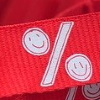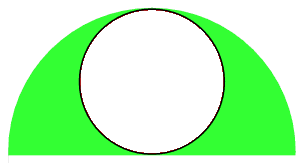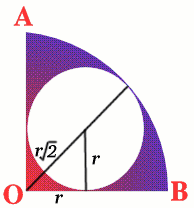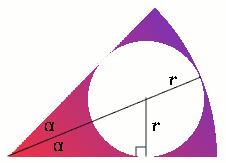#### You may also like### Percentage Unchanged

If the base of a rectangle is increased by 10% and the area is unchanged, by what percentage is the width decreased by ?### Circle Packing

Equal circles can be arranged so that each circle touches four or six others. What percentage of the plane is covered by circles in each packing pattern? ...### Fractions and Percentages Card Game

Can you find the pairs that represent the same amount of money?

# Round and Round

##### Age 14 to 16 Challenge Level:

Well done Kang Hong Joo from the Chinese High School, Singapore; Lucinda Hearth from Stamford High School; Jessica Zhang; Matthew Hodgetts from King Edward VI Camp Hill School, Birmingham; Tom Davie and Michael Grey from Madras College, St. Andrews.

Case 1: a semicircleLet the radius of the inner circle be $r$; then its area is $\pi{r^2}$. The area of the semicircle is $\pi(2r)^2/2$, which is $2\pi{r^2}$. The percentage of the semicircle covered by the inner circle is 50\%.

Case 2: a quadrantThe area of the inner circle is $\pi{r^2}$; the radius of the quadrant is $r(1 + \sqrt{2})$, and the area of the quadrant is $$\frac{1}{4}\pi{r^2}(1 + \sqrt{2})^2 = \frac{1}{4}\pi{r^2}(3 + 2\sqrt{2})$$ Therefore $$\frac{\text{area of inner circle}}{\text{area of quadrant}} = \frac{4}{3 + \sqrt{2}} = 68.6\%$$

Case 3: a sector of angle $2\alpha$The area of the inner circle is $\pi{r^2}$. Taking the radius of the sector to be 1 unit, then $$\sin\alpha = \frac{r}{1 - r}$$ Hence $$r = \frac{\sin\alpha}{1 + \sin\alpha}$$ The area of the sector is ${1\over2} 1^2 (2a) = a$ or, equivalently, ${\pi{\alpha}\over180}$ if $\alpha$ is measured in degrees.

Therefore $$\frac{\text{area of inner circle}}{\text{area of sector}} = \frac{\pi\sin^{2}\alpha}{\alpha(1 + \sin\alpha)^2}$$ When $\alpha = {\pi\over6} = 30^{\circ}$, then $\sin\alpha = {1\over2}$, and this ratio is $2/3$.

When $\alpha = {\pi\over3} = 60^{\circ}$, then $\sin\alpha = {\sqrt{3}\over2}$, and this ratio is $$\frac{3\pi/4}{(\pi/3)(1+\sqrt{3}/2)^2} = \frac{9}{2+\sqrt{3}} = 9(2 - \sqrt{3})^2 = 64.6 \%$$
 a 30 o 45 o 60 o 90 o Ratio (nearest 1%) 67% 69% 65% 50%